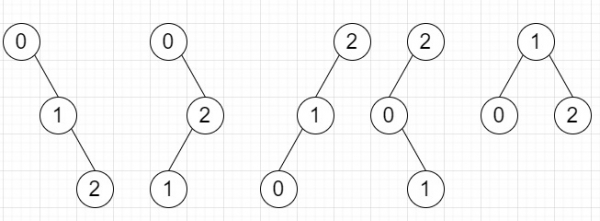# Program to count number of unique binary search tree can be formed with 0 to n values in Python

Suppose we have one number n, we have to find the number of unique BSTs we can generate with numbers from [0, n). If the answer is very large mod the result by 10^9+7

So, if the input is like n = 3, then the output will be 5To solve this, we will follow these steps −

• numer := 1
• denom := n + 1
• for i in range 1 to n, do
• numer := numer * n + i
• numer := numer mod m
• denom := denom * i
• denom := denom mod m
• numer := numer * (denom^(m-2)) mod m
• return numer mod m

Let us see the following implementation to get better understanding −

## Example

Live Demo

class Solution:
def solve(self, n):
m = 10 ** 9 + 7
numer = 1
denom = n + 1
for i in range(1, n + 1):
numer *= n + i
numer %= m
denom *= i
denom %= m
numer *= pow(denom, m-2, m)
return numer % m
ob = Solution()
print(ob.solve(4))

## Input

4

## Output

14